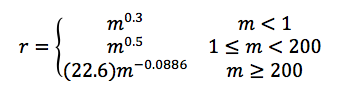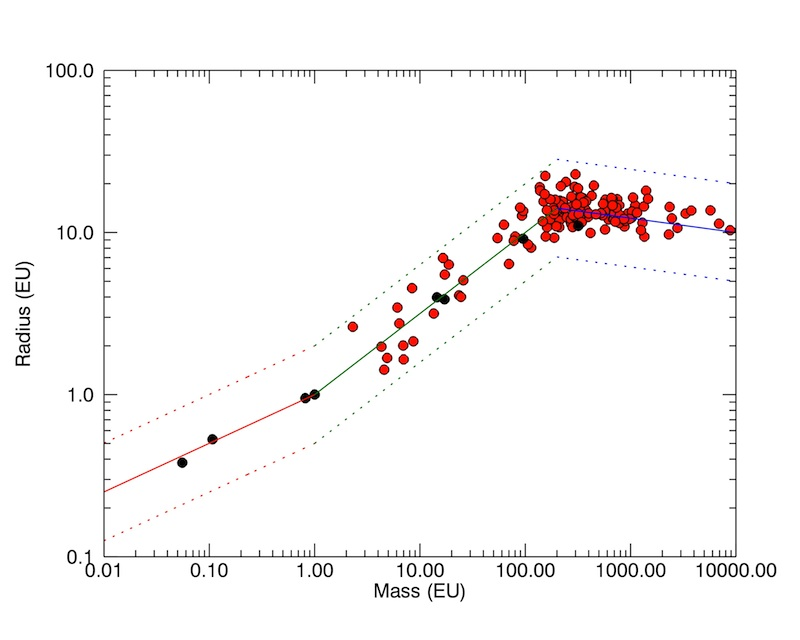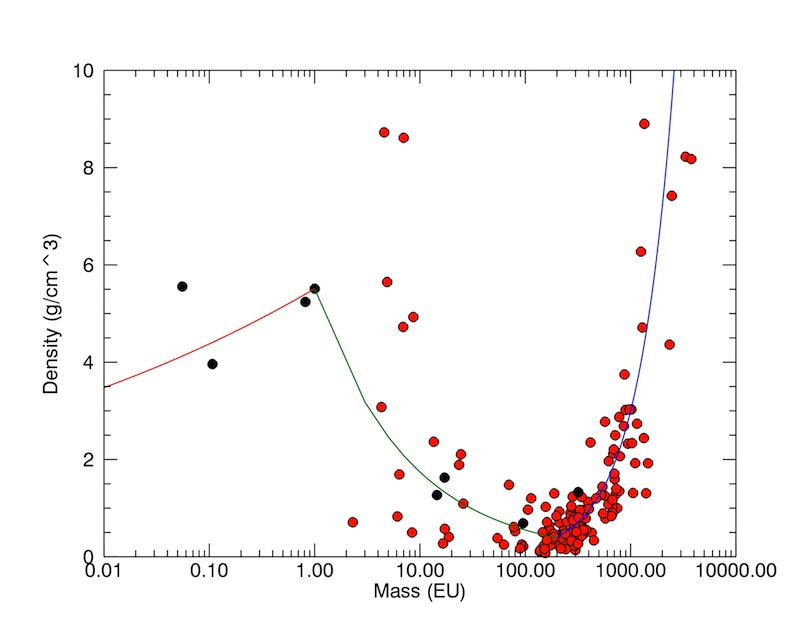Library‎ > ‎LabNotes‎ > ‎

### Standard Mass-Radius Relation for Exoplanets

posted Dec 14, 2011, 3:56 PM by Abel Mendez   [ updated Jun 30, 2012, 11:54 AM ]
 We are trying to develop a standard mass-radius relationship for exoplanets to predict the most likely value from the other. There are very high uncertainties in predicting radius from mass but we just need a reference relation. Here we created a simple empirical formulation combining solar and extrasolar planets data. The relation assumes that any body below one Earth mass is rocky in composition, those between 1 to 200 Earth masses start as rocky but move quickly to oceanic and gas giants, and those above 200 Earth masses are gas giants (Figure 1 and 2). The relation is give by:where m is mass in Earth masses and r is radius in Earth radii. This relation is very simple but will probably be updated with a better one in later posts.Figure 1. Empirical mass-radius relation for exoplanets. The dotted lines show two and half times the predicted value, all fall within this maximum error boundary. The data is from confirmed exoplanets with mass and radius. EU = Earth units.Figure 2. Density vs mass for the mass-radius relation shown in figure 1.